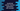# JavaScript substr() function explanation with example# Introduction :

The substr function is used to get one substring from a string in Javascript. In this example, we will learn how to use the substr method in Javascript with examples.

## Syntax :

The syntax of the substr method is as below :

``str.substr([start , length])``

## Arguments and return value :

This method takes two arguments :

start : This is the start index from where the substring is required.

length : The length of the substring. If this value is not provided, it returns the substring to the end of the string.

### Example :

If we call the substr method without start and length, it will return the same string. For example :

``````    let str = "abcdefghijklmnopqrstuvwxyz";

console.log(str.substr());
console.log(str.substr(5));
console.log(str.substr(5,5));``````

### Output :

The above program prints the below output :

``````    abcdefghijklmnopqrstuvwxyz
fghijklmnopqrstuvwxyz
fghij``````

### Explanation :

In the above example,

The first console.log statement prints the whole string because we are calling substr method without start and length.

The second console.log statement prints one substring to the end starting from the index 5.

The third console.log statement prints one substring of size 5 starting from the index 5.

### Conclusion :

In this example, we have learned how to use substr method in Javascript to get one substring of a string. Try to go through the examples above and drop one comment below if you have any queries.